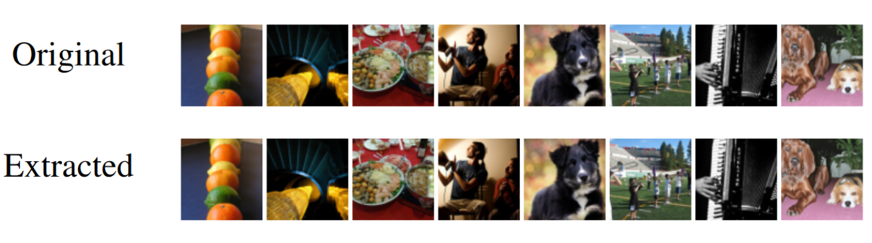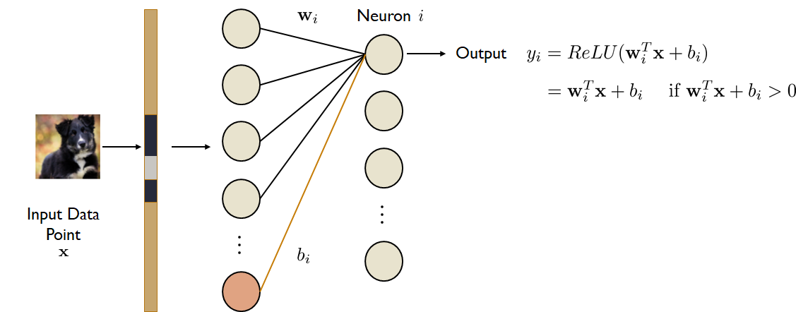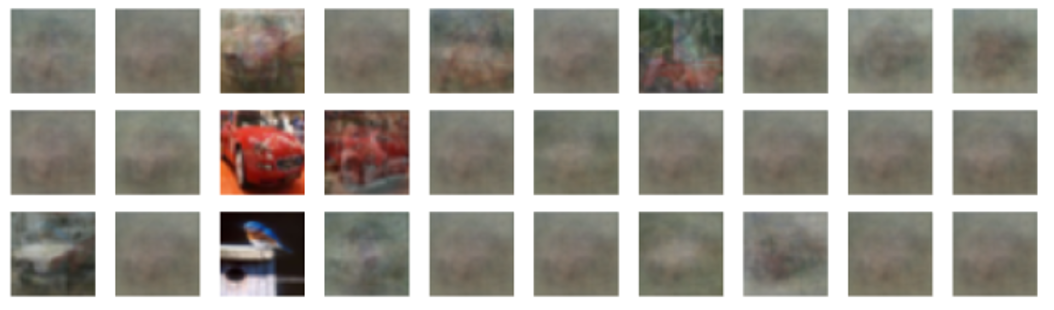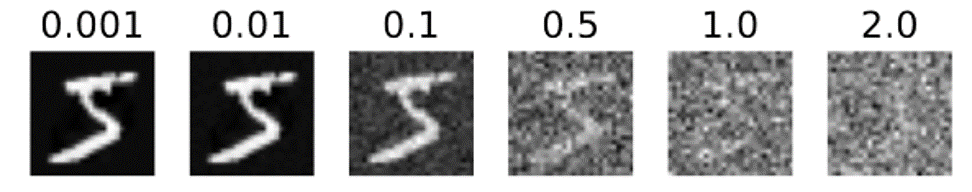### or Federated Learning with Untrusted Servers is Not Private

by Franziska Boenisch, Adam Dziedzic, Roei Schuster, Ali Shahin Shamsabadi, Ilia Shumailov, and Nicolas Papernot

Every day, we all generate large amounts of data such as the text we type or the pictures we take. This data is useful: by training machine learning (ML) models on data collected from millions of individuals, a myriad of applications become possible, a prominent example of which is text auto-completion by our smartphone’s keyboard.

However, how can we collect such large amounts of data from so many users at one central server, given the communication overhead and the central storage that would be needed? And how much computational power would we need to train an ML model on all of the collected data points? And even if we could overcome the onerous communication, computation and storage requirements, do we really want to transmit all this highly personal data to a single central party which could be the smartphone vendor in our keyboard auto-completion example?

In a seminal paper from 2017, a framework called Federated Learning (FL) was proposed to answer these concerns [McMahan et al.]. In Federated Learning, training is performed locally on device using the individual users’ data. The products of this local training are then sent to a server, where they are aggregated from multiple devices into a global model. The server never receives the raw user data, only the products of the local training procedure (let’s call them gradients or model updates).

Since users’ data never leaves devices, FL has, for a long time, been promoted as “privacy-preserving ML”. Today — thanks to a long line of research — we know that model updates actually contain ample information on individuals’ data and allow full reconstruction of their data in some cases, meaning that FL cannot actually be considered privacy-preserving. However, large companies did still promote FL as privacy-preserving and deployed it. One way to explain why that is the case is two widely-adopted beliefs: first, that reconstruction methods are computationally costly, and typically obtain low-fidelity reconstructions, especially for data that is high dimensional, or contains multiple instances from the same class, or when the local gradients are calculated over many user data points. Second, that FL combines well with protocols that make reconstruction attacks significantly harder or even provably impossible, namely, (local) Differential Privacy and Secure Aggregation.

Our new work questions both of these widely-held beliefs through perfect reconstruction of data with near zero costs. Our main message is that, as of yet, FL provides no viable way to defend against a non-honest (“malicious”) server. This means a server that intentionally deviates from the FL protocol as prescribed, in order to perform the attack. In other words, to get privacy, we still must trust the party that is responsible for FL deployment (e.g. our smartphone vendor).

We first show an attack where the central server actively manipulates the initial weights of the shared model, to directly extract user data perfectly, i.e. with zero error to the original data. Our attack is the first privacy attack in FL that relies on manipulations of the shared model. Notably, we find that, depending on the deployment of the FL setup, our extraction procedure works even in the honest setting without the server maliciously initializing the weights. Even in this setting, the server can exactly reconstruct user data instances in a matter of milliseconds, defeating the state-of-the-art in passive reconstruction attacks by orders of magnitude. To illustrate this, here are pictures extracted by our attacker, armed with only a Python shell and Matplotlib.Images from the ImageNet Dataset, extracted from the user gradients. Extraction is perfect, i.e., the error between the original and respective extracted images is zero.

This result underscores that FL in its naive, “vanilla” form does not provide privacy when the server is untrusted.

But what about Secure Aggregation (SA) and Differential Privacy (DP), you ask? Well, it was recently observed by [Pasquini et al.] that SA can lose its guarantees when the server can send different models to different users. In other words, it is not secure against a malicious server, just like FL itself. Worse yet, virtually all SA protocols we are aware of rely on a proportion of participants being honest. In practical scenarios, if the party deploying the protocol, acts maliciously, even for only short periods of time, they can invoke arbitrarily many manipulated participants (“sybils”) in the FL protocol. Thereby, they can completely circumvent the protection offered by SA. This ability of introducing manipulated participants in real-world FL systems has been shown [Ramaswamy et al.]. Under a malicious-sybil regime, it is likely that distributed DP also fails: a user cannot rely on other users’ updates being properly DP-noised, and must compensate by noising their own updates so much that learning would not likely be possible. While “hybrid” protocols that interleave elements of both SA and DP might address these issues (which we will discuss in greater depth), right now they are currently far from being practical enough to be widely adopted.

Below, we go into the nitty-gritty details of our attack. We will first focus on passive data leakage from gradients (without malicious weight initialization), and then explain our active, malicious-server attack.

To conclude on a positive note, we will discuss some promising directions for mitigating this and other attacks, such as hybrid SA-DP and blockchain-based methods for ensuring server accountability and model cohesion across participants. We believe that federated learning, when combined with secure aggregation and differentially private training, can provide some form of privacy as long as the user has sufficient trust in the central server.

## Directly Extracting Data from Model Gradients (Passive Leakage)

Let’s first have a look at the passive data leakage from model gradients. This leakage can be exploited even by an honest-but-curious central server who observes the user gradients, calculated on a fully-connected neural network layer.

### Why is One Input Data Point Perfectly Extractable from Model Gradients?

Previous work had already shown that gradients, when calculated for one single input data point $$\mathbf{x}$$ at a fully-connected model layer contain a scaled version of this input data point. This holds if the fully-connected layer has a bias term, uses the ReLU activation, and at least for one row $$i$$ in the layer’s weight matrix, the product of the input data point and this row, plus the corresponding bias, is positive. The setup can be visualized as follows:Setup of propagating a data point x through a fully-connected layer.

The reason why the data point $$\mathbf{x}$$ can be extracted from the gradients of the layer’s weight matrix at row $$i$$ can be explained by simply using the chain rule in the calculation of the gradients.

(1) $$\begin{equation} \frac{\partial \mathcal{L}}{\partial b_i} = \frac{\partial \mathcal{L}}{\partial y_i} \frac{\partial y_i}{\partial b_i} \end{equation}$$

(2) $$\begin{equation} \frac{\partial \mathcal{L}}{\partial \mathbf{w}^T_i} = \frac{\partial \mathcal{L}}{\partial y_i} \frac{\partial y_i}{\partial \mathbf{w}^T_i} \end{equation}$$

In addition, for $$\mathbf{w}^T_i \mathbf{x} + b_i > 0$$ we know: $$y_i = \mathbf{w}^T_i \mathbf{x} + b_i$$, and, $$\frac{\partial y_i}{\partial b_i} = 1$$, due to the derivative calculation.

So we can add this latter term to the previous equation (1) and obtain the following: $$\begin{equation} \frac{\partial \mathcal{L}}{\partial b_i} = \frac{\partial \mathcal{L}}{\partial y_i} \frac{\partial y_i}{\partial b_i} = \frac{\partial \mathcal{L}}{\partial y_i} \end{equation}$$

If we input $$\frac{\partial \mathcal{L}}{\partial y_i}$$ in the other equation (2), we end up with $$\begin{equation} \frac{\partial \mathcal{L}}{\partial \mathbf{w}^T_i} = \frac{\partial \mathcal{L}}{\partial y_i} \frac{\partial y_i}{\partial \mathbf{w}^T_i} = \frac{\partial \mathcal{L}}{\partial b_i} \mathbf{x}^T \end{equation}$$

At this point, it is obvious that the gradients contain a scaled version of the input data point $$\mathbf{x}$$. It is scaled by the factor $$\frac{\partial \mathcal{L}}{\partial b_i}$$, which is nothing more than the gradient w.r.t. the bias. And this gradient is sent from the user to the server in FL, hence, the server can simply reverse the scaling of $$\mathbf{x}$$, by multiplying with the inverse of $$\frac{\partial \mathcal{L}}{\partial b_i}$$, i.e. $$\frac{\partial \mathcal{L}}{\partial b_i}^{-1}$$, if $\frac{\partial \mathcal{L}}{\partial b_i} \ne 0$.

### Why are Individual Data Points still perfectly Extractable from Gradients of Large Mini-Batches of User Data?

Usually, gradients are not calculated over one individual input data point though, but rather on a larger mini-batch consisting of several input data points. The mini-batch gradients $$G_{total}$$ represent an average over all individual data points’ gradients over the entire mini-batch. If there are $$B$$ data points $$\mathbf{x}_j$$ in the mini-batch, then the total gradients can be calculated as follows: $$G_{total}=\frac{1}{B} \sum_{j=1}^B G_{x_j}$$. Given this, we expect the rescaled gradients to look like an average over all data points in the mini-batch.

However, surprisingly, when we use matplotlib to depict some rescaled gradients of a mini-batch consisting of 100 data points from the CIFAR10 dataset, we are still able to identify some of the individual data points:Rescaled gradients of a mini-batch of 100 data points from the CIFAR10 dataset. Gradients correspond to the first 30 weight rows at the first fully-connected layer of a randomly initialized fully-connected neural network. We observe that some input data points are perfectly extractable.

This behavior can be explained by revisiting the properties of the ReLU activation function. If the input $$\mathbf{w}_i^T\mathbf{x}+b_i$$ at neuron $$y_i=ReLU(\mathbf{w}_i^T\mathbf{x}+b_i)$$ is negative, the ReLU function outputs zero. In this case, no information about this data point $$\mathbf{x}$$ is propagated through the neural network at this neuron. With no information about this data point, the corresponding gradients are zero. If this happens for all but one data point $$\mathbf{x}_m$$ in a mini-batch, the average gradients correspond to $$G_{total}=\frac{1}{B} \sum_{j=1}^B G_{x_j} = \frac{1}{B} (0+\dots+0+G_{x_m}+0+\dots+0)$$. In this case, the average gradients only contain the gradients of one single data point $$\mathbf{x}_m$$, and we have seen that this data point is perfectly extractable.

Our experiments show that the percentage of individual user data points which can be perfectly extracted from the gradients depends on two main factors:

• Mini-batch size: the fewer data points we have in a mini-batch, the higher the percentage of extracted data points. This is because there are fewer data points that can potentially overlay.
• The number of neurons at the fully-connected layer: the more neurons we have, the more “chances” there are to have the situation in which the input to the neuron is positive only for one data point from the mini-batch.

To provide concrete examples, we found that even for complex datasets, such as ImageNet, when we have as many as 100 data points per mini-batch, and as little as 1000 neurons at the first fully connected layer, we can extract roughly 21 original data points (out of 100) perfectly.

## Active Attacks on the Shared Model Weights

Based on the insights about the data leakage from gradients, we explored how a malicious server who can manipulate the weights of the shared model is able to amplify the leakage of individual user data points and even to extend our attack to convolutional neural networks.

To increase the leakage and corresponding extraction success, we propose to initialize the model weights with a method we call trap weights. Our trap weights aim at deliberately causing the inputs to neurons and their ReLU activation function to be negative for most input data points. We do so by scaling down the positive components in the fully-connected layer’s weight matrix. Thereby, the negative values gain a larger influence in the product with the input data points. This increases the chance that very few (in the best case only one) input data point from a mini-batch produces positive input to the neuron. In that case, the data point is perfectly extractable.

For our trap weights, we do not require particular background knowledge on the data distribution, and the only assumption we make is that the input data is scaled to the range [0,1], which is a common pre-processing step. Our results indicate that our trap weights increase the extraction success significantly. In the ImageNet example, more than twice as many individual data points can be extracted (now 45 instead of 21 in the same setup). Similar to the passive extraction attack, the percentage of perfectly extractable data points increases with smaller batch sizes and more neurons at the layer.

We also propose an adversarial initialization of the model weights to make our attack applicable when there are some convolutional layers before the first fully-connected layer where we can extract the data. To do so, we convert the convolutional layers into identity functions transmitting the input data points to the first fully-connected layer. This transmission is possible if, at every model layer’s feature maps, there are enough parameters to accommodate all features of the input data. Since convolutional neural networks usually shrink the input’s width and height but increase the depth, there exist architectures where this is the case, namely when the architecture makes sure that at every layer, there are as many parameters as input features. Our active attack, therefore, also works for certain convolutional neural network architectures.

The results of the data extraction after our adversarial weight initialization are displayed in the following figure:Reconstruction success of our adversarial initialization: all reconstructed data points from a mini-batch of 100 ImageNet data points, extracted at the first fully-connected layer in a convolutional neural network. Gray images indicate that the corresponding original data point could not be extracted perfectly from the model gradients.

Also note, that the server does not need to adversarially initialize the shared model at every iteration of the protocol, or for every user. Instead, it can execute the normal FL protocol for most of the time, and only at some iterations of the protocol send out a manipulated model to some specific target users. This does not only help to train a useful shared model the rest of the time and with the non-target users, but also renders the attack more inconspicuous at the users’ end. This is because most users will receive a valid model, and the target users who receive a maliciously initialized model have no means to verify whether this model is manipulated, or is just the product of previous model update steps among other users.

## Is it possible to Prevent Our Passive and Active Attacks?

There are several extensions of FL which aim at preventing attacks like ours, the most prominent ones are Secure Aggregation (SA), training with Differential Privacy (DP), or a combination of both.

### Secure Aggregation

When introducing SA into the FL protocol, the server is no longer in charge of aggregating the different user gradients. Instead, the users apply a form of secure multi-party computation to jointly aggregate their gradients and share them with the server only afterwards. This decreases privacy leakage from the gradients since the gradients of more data points are then overlaid. Additionally, if the server still manages to extract some individual data points, it does not know what user this data came from.

### Differential Privacy

A standard framework to implement privacy is differential privacy (DP). DP algorithms allow the disclosure of statistical information about some data without revealing information about particular individuals in the data.

There are different ways to implement DP in FL, such as (1) a distributed version of the standard DP-SGD [Ramaswamy et al.] in which the users clip their gradients and the central server adds noise, (2) local DP [Truex et al.] in which each user adds large amounts of noise to their local gradients, and (3) distributed DP [Kairouz et al.], and [Agarwal et al.] that relies on each user adding small amounts of local noise that provide meaningful privacy guarantees only when being aggregated before sending them to the server. (1) falls short when acknowledging the possibility of a malicious server who cannot be trusted with the noise addition. (2) has been shown to drastically degrate the utility of the shared model [Kairouz et al.]. (3) can be implemented, for example, with SA, however, even when a single user fails to add the noise, the privacy guarantees do not hold any longer.

## Trust is All You Need

Unfortunately, when dealing with a malicious central server, even (3) cannot prevent our attacks. This is because, as we mentioned in the introduction, SA in FL can be eluded as shown by [Pasquini et al.] when the server sends out different models to different users. Alternatively to this approach, a malicious server could also directly control a very small fraction of devices that participate in the FL protocol, select them to participate in the SA together with one target user’s device, and make the controlled devices contribute only zero gradients to extract the target user’s private data from the aggregated gradients. In practice, it is difficult for an end user of FL to know when the central server orchestrating the FL protocol selected sybil devices to participate with the end user’s device. Put another way, the end user has to assume the central server will not try to circumvent SA, which assumes a fraction of honest participants.

On the face of it, it appears like users can add differentially-private noise locally (referred to as local DP), to protect their update even if it is not aggregated with anything before it is received by the server. Unfortunately, as we qualitatively show, adding sufficient noise is likely to result in devastating utility losses for the resulting model.Quality of the extracted data, depending on the noise scale of the Gaussian noise added to a data point from the MNIST dataset.

Given the above discussion, we recommend that users who do not wish to reveal their data to the FL orchestrator do not participate in the protocol.

## What can a central server do to implement meaningful privacy in FL?

Based on our attacks, we can consider some best practices and directions for how untrusted central servers could still implement FL as privately as possible:

1. Ensure that user gradients/updates are calculated over large mini-batches of local data.
2. Use shared models with architectures where our attack is more difficult (e.g. containing aggressively lossy layers such as Dropout or Pooling).
3. Use secure computation, or a hardware-based trusted execution environment, to initialize the model weights and provide proof/attestation that they were initialized following standard practice.
4. Commit to the shared model’s weights in a publicly accessible trusted location, for example, on a distributed public ledger (blockchain). Users can then verify that their local shared models are consistent with the public commitment, thus ensuring coherence across users.
5. Devise a (maliciously-) secure multiparty computation mechanism where DP noise is added as a part of the aggregation procedure, and the amount of noise is commensurate to the number of participants. As we explained above, to provide protection in most practical settings, the protocol must provide a meaningful guarantee even if all but a single participant are malicious, regardless of the number of participants.

Applying all these measures together, perhaps, can make FL “privacy-preserving” in a strong sense, and helps users to protect their sensitive local data. As of yet, however, the computational and utility costs of these enhancements are still to be quantified.